## Bijections and counting

### Quick description

There is a bijection between two finite sets if and only if they have the same number of elements. Many [seemingly] difficult to count sets can easily be counted by mapping them bijectively to sets which are [seemingly] simpler to count.

A bijection can also be referred to as a change of variable.

This is a very general idea and the main problem solving technique it suggests is this: if a set appears difficult to count, try to find a representation of it that is easier to count.

### Prerequisites

Basic mathematical notation.

### Example 1

(William Feller, Probability Theory, Vol I, Third edition). Let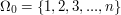be a finite set and let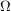be unordered strings of length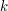from this alphabet. For a string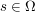, let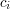be the number of times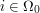appears in. We can representwith a distribution ofidentical balls intocells by puttingballs into cell. Each of these distributions, in their turn, can be uniquely represented by a string of length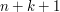from the alphabet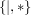with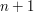's and's and which start and end with. The's represent the identical balls and the's the walls of cells. Let's denote the set of all strings of this form with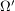.

Here is an example: for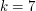and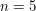the string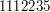is represented by the string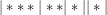It is clear thatandcan be mapped bijectively.is very simple to count using multiplication and partitioning: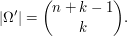Therefore, we have that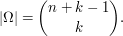### Example 2

The reflection principle. (William Feller, Probability Theory, Third Edition, Chapter III). Let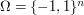. Let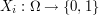be the coordinate maps. Define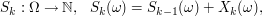for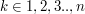and and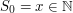. For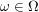,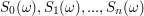can be thought of as a trajectory of a process taking values in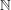, which starts from, and at each discrete time step goes either up or down by.

Let us fix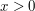and consider the set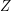of trajectories that end up at position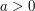at their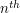step which also visit the origin in their excursion. In other words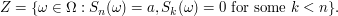Knowing the size of this set allows one to compute, among other things, the distribution of the first hitting time to a state as well as the last exit from a state of a symmetric random walk. The weak limits of these distributions under proper scaling gives the distributions of the same random variables for the Brownian motion. These in turn can be used to compute distributions of analogous quantities for more complicated processes derived from these simpler ones. Thus knowing the size of the setis useful and interesting. However, defined as is, the set is not simple to count because it involves a complicated constraint (at least to the human mind), i.e., that each trajectory being counted has to hit the origin. We will now mapbijectively to a simpler set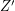with no constraints and which is simple to count.

We set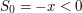, and defineto be the set of trajectories that hit levelat step.is a set of the same type as, except that there are no constraints on it.

Now let us define the bijection that will mapto. Let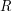be the map that reflectsalong the time axis upto the first time it hits the origin. That is, for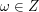letbe the first time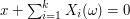and defineas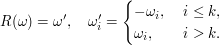The following figure shows the action ofon a sample path.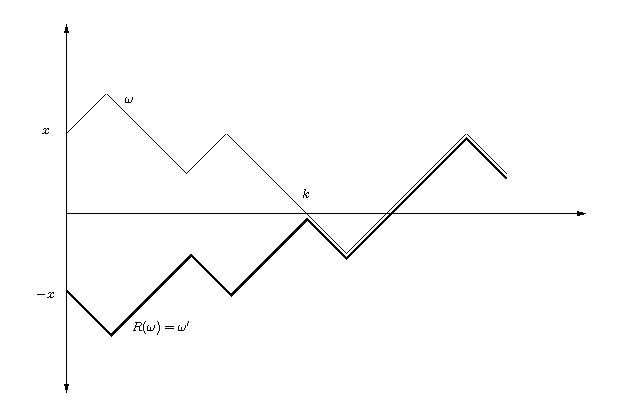It follows directly from its definition thatis a bijection fromto.

One can countas follows. For any path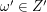let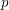be the number of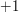steps andbe the number of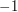steps in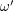. By's definitionandis the same for all paths inand they satisfy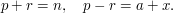The number of paths inis then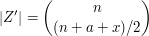if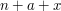is even, and zero otherwise. Becauseis bijectively mapped towith, the binomial coefficient in the last display also gives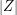.

### General discussion

Using a change of variable to simplify a counting problem is a special case of the general idea of using a change of variable to translate one problem to another.

The following comments were made inline in the article. You can click on 'view commented text' to see precisely where they were made.

### Surely you are counting

Surely you are counting unordered strings here?

### ordered/unordered

Thanks for the comment and sorry for the confusion. What I meant was "sorted". We can use `unordered', if "sorted" is confusing/ not standard. Thanks again.

The following comments were made inline in the article. You can click on 'view commented text' to see precisely where they were made.

### Incorrect Image

The image of each X_i ought to be {-1, 1}, I think.

## Post new comment

(Note: commenting is not possible on this snapshot.)

Before posting from this form, please consider whether it would be more appropriate to make an inline comment using the Turn commenting on link near the bottom of the window. (Simply click the link, move the cursor over the article, and click on the piece of text on which you want to comment.)